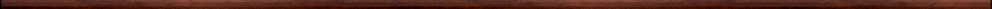Getting started with MapleIntroductory, general and miscellaneous worksheets:

They are all compatible with Classic Worksheet Maple 10.Maple Basics - basics.mws

• Exact numerical calculations: *, ^ , ifactor and sqrt.
• Floating point arithmetic with Maple and assignment: evalf, :=, Digits.
• Plotting graphs: plot, seq.
• Expressions and functions ->, eval.
• Solving equations: solve and factor.
• Sample graphs.
• Differentiation and integration: diff, int, Diff, Int.
• Some algebraic manipulations: expand, normal, simplify
• The limit of sequence and the limit of a function: limit, Limit.
• The sum of a series: sum, Sum
• Maple is just a tool

The next worksheet is adapted from worksheets designed by the Mathematics Department at Seattle Central Community College. The original worksheets can be downloaded from the Maple website:  Maple Tutorial

Maple essentials tutorial - tutorial.mws

• Introduction to the tutorial.
• Section 1: Numerical calculations.
• section 2: Algebraic calculations.
• Section 3: Plotting graphs.
• Section 4: Solving equations.
• Section 5: Defining and evaluating functions.
• Section 6: More on graphing.
• Notes on the Maple interface.
• Quick reference for Maple commands.
• Practice problems

The next worksheet is adapted from a worksheet by A. Kluge, The University of Texas at Austin http://www-math.cc.utexas.edu/math/Maple

Getting started with Maple - start.mws

• Introduction
• Launching Maple
• How to use this worksheet
• Simple uses of Maple
• Defining functions
• Maple plots
• Getting help
• Differentiation and integration
• Solving equations
• Matrices and linear equations
• Closing thoughts
• Glossary of Maple symbols

The next two worksheets are based on worksheets by Carl Eberhart, Dept. of Mathematics, University of Kentucky http://www.ms.uky.edu/~carl

What is Maple? - whatis.mws

• Four Properties of Maple.
• The Worksheet: A handy place to solve problems.
• Get to know the language.
• Experiment!

A short introduction to the Maple language - intro.mws

• Arithmetic
• Expressions, names, statements, and assignments
• Functions
• Built-in Maple functions and composition of functions
• Using Maple as a fancy graphing calculator
• Data types: expression sequences, lists, sets, arrays, tables
• Maple control statements
• A brief vocabulary of Maple words
• Trouble shooting notes

Programming in Maple . . first steps - prog.mws

• Automating commands
• Factorials . . an example of a Maple procedure
• Fibonnacci numbers
• Conversion to binary
• Plotting graphs of several functions

Top of page

Main index